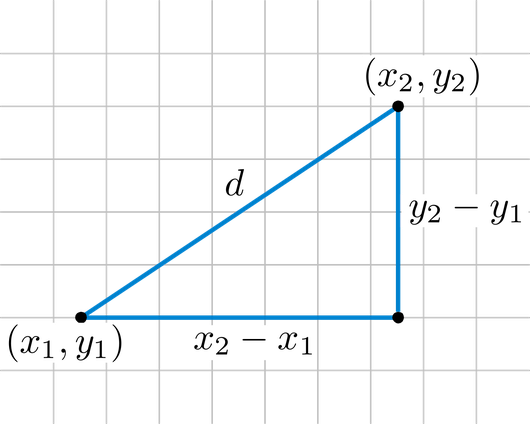# Glossary

## Euclidean distance

The Euclidean distance between two points in either the plane or 3-dimensional space measures the length of a segment connecting the two points. It is the most obvious way of representing distance between two points.

The Pythagorean Theorem can be used to calculate the distance between two points, as shown in the figure below. If the points $(x_1, y_1)$ and $(x_2, y_2)$ are in 2-dimensional space, then the Euclidean distance between them is $\sqrt{(x_2 - x_1)^2 + (y_2 - y_1)^2}$.For points $(x_1, y_1, z_1)$ and $(x_2, y_2, z_2)$ in 3-dimensional space, the Euclidean distance between them is $\sqrt{(x_2 - x_1)^2 + (y_2 - y_1)^2 + (z_2 - z_1)^2}$. For example, the Euclidean distance between $(-1, 2, 3)$ and $(4, 0, -3)$ is $\sqrt{25 + 4 + 36} = \sqrt{65}$.